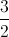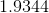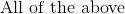# High School Math : Understanding Real Numbers

## Example Questions

### Example Question #1 : Number Theory

Without using a calculator, which of the following is the best estimate for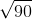?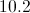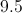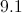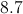Explanation:

We know that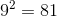and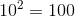.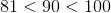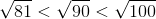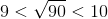Because 90 falls is approximately halfway between 81 and 100, the square root of 90 is approximately halfway between 9 and 10, or 9.5.

### Example Question #2 : Number Theory

Place in order from smallest to largest: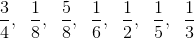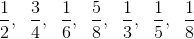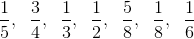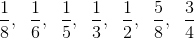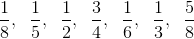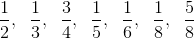Explanation:

To place in order, first we must find a common denominator and convert all fractions to that denominator.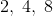have a common denominator of.have a common denominator of.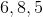have a common denominator of.

Therefore we can use common denominators to make all of the fractions look similar.  Then the ordering becomes trivial.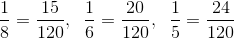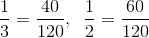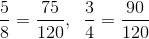### Example Question #3 : Number Theory

What number isof?Explanation:

For percent problems there are verbal cues:

"IS" means equals and "OF" means multiplication.

Then the equation to solve becomes: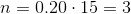### Example Question #4 : Number Theory

Which of the following is NOT a real number?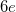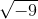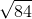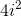Explanation:

We are looking for a number that is not real., andare irrational numbers, but they are still real.

Then,is equivalent toby the rules of complex numbers. Thus, it is also real.

That leaves us with:which in fact is imaginary (since no real number multiplied by itself yields a negative number) and simplifies to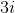### Example Question #5 : Number Theory

Which of the following are considered real numbers?